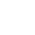## Wednesday, February 5, 2014

### FSE : Defining optimal subranges

Note : Charles Bloom started an independent in-depth analysis of ANS on his blog, which is definitely worth the read.

As stated in earlier FSE comparison with Arithmetic Coding, FSE deals with fractional bits by "decreasing" its state value. Now how does that work precisely ?

Let's use again the example of a symbol which probability of appearance is 5/4096. The Shannon limit for this symbol is 9.68 bits per symbol (if we do better, then some other symbols will suffer, and the overall will compress less). As stated earlier, we deal with the fractional bit issue by outputting sometimes 9 bits, sometimes 10 bits.

The distribution of 9-bits & 10-bits sub-ranges must ensure that all possible values remain accessible after the current symbol. It's a condition for lossless compression. The result is the following layout :

OK, so why are the 9-bits subranges positioned at the beginning of the range ?
Well, in previous article, we said that an additional bit is read when the state is so much decreased that is goes below lower limit. In which case, it just "wraps around", starting again from the top value, at the cost of an additional bit read.

So it's not so much that the 9-bits are at the bottom. Rather, with above explanation, it is more intuitive to explain that the 10-bits are at the top.
Maybe it would be easier to notice with another example, a symbol with 7/4096 probability of appearance.

Note that these subranges are "destination ranges". Each of the 7 symbol_cells are mapped to one and only one of these ranges.

So which symbol is associated with each range ?

As stated earlier, since the additional bit is "paid for" when crossing the low limit, the most likely symbol to trigger the limit is lowest one.
So we give an index to each of the 7 symbol_cells, in their order of appearance, from the smaller to the larger one.
The largest 10-bits range is attributed to symbol_cells n°1.
The other ranges simply follow, in their natural order :

This is coherent with an optimal cost associated with a 7/4096 distribution : 9.19 bits.
It means that we are only consuming 0.19 fractional bits each time the symbol is decoded. So we can decode it about 5 times at 9-bits before requiring 10-bits.

This is in contrast with a probability of 5/4096 :

Here, the optimal cost is 9.68 bits, so fractional bits is much higher (0.68 bits). We are likely to cross the low limit much more often. Hence the first 3 symbol_cells trigger the additional bit read, and require 10 bits.

This rule is valid for any probability; so now, whether it is 5/4096, 134/4096, or even 3812/4096, you know how to define destination sub-ranges, and can build the associated decoding table.
(The latest example is interesting, since some sub-ranges will be 0-bits large, which means they map directly to another state value : 3812/4096 => 284 1-bit sub-ranges + 3528 0-bit sub-ranges).

What remains to be understood is how to distribute the symbol states. A naive approach would be to simply cluster them together. For example, a symbol with probability of 7/4096 would occupy state values from 0 to 6. It would work, but compression effectiveness would be very poor.
In another blog post, we'll see why, and how to correct that.

1.Thank you for your kind explanation.
However, some posts are hard to follow up due to missing images.
Most of all drop box images are missing in your post.
It would be great to understand better with missing images.

1.That's a good point.
Dropbox changed its policy regarding image hosting,
to force users to go through their own webpage (with login, ads, and such).
I'll see what I can get back from there.

2.This is fixed. Images are now hosted on blogspot directly, so no more direct access issue.
For some reason though, image rescale is somewhat more fuzzy than it used to.
Click the image to get full view if it's a problem